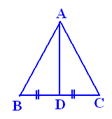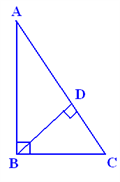# Triangles

Triangles are one of the most common figures in geometry. Questions related to triangles are very common in the CAT quantitative aptitude section. Here is a detailed material on triangles that can help the CAT aspirants to prepare this topic effectively.

### Definition of a Triangle:

Triangle is a polygon which has three edges and three vertices, the sum of all the angles of a triangle is 180º.

### Classification of Triangles:

Triangles can be classified according to the size of its angles. Some examples are right triangles, acute triangles, and obtuse triangles.

The lengths of the sides of triangles is another common classification for types of triangles. Some examples are equilateral triangles, isosceles triangles, and scalene triangles.

### Properties of Triangles:

In triangle ABC,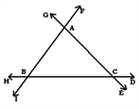1) Sum of Interior angles = 1800

2) Sum of Interior angle+adjacent exterior angle= 1800

3) Measure of exterior angle= Sum of two interior angles not adjacent to it (<ABH=<BAC+<ACB)

4) If two sides of a triangle are not congruent, then the angle opposite the greater side is greater. The converse also holds true.

5) Sum of any two sides of a triangle is greater than the third side; i.e. if the sides are a,b and c then a+b>c

6) Difference between any two sides of a triangle is lesser than the third side, i.e. a-b.

### Is the point of intersection of the Altitudes.

Altitude:- A perpendicular drawn from the vertex of a triangle to the opposite side (the base) of the triangle is called the altitude of the triangle.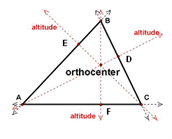In the diagram;

AD, CE, and BF are all altitudes.

### It is the point of intersection of the three medians

Median:- A median is drawn from the vertex of a triangle divides the opposite side into 2 equal halves. It also divides the area of the triangle into 2 equal halves.

The centroid divides each median in the ratio 2:1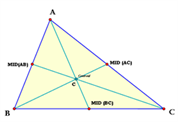In the triangle ABC; AX, BY and CZ are all medians.

### It is the point of intersection of perpendicular bisectors

Perpendicular Bisector( bisector):- This divides the side of the triangle into two equal halves and is also perpendicular to it.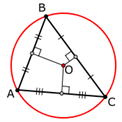In the triangle O is the circumcenter

Angle Bisector:- This divides the angle from which it is drawn into two equal halves.

#### 4) Incentre:

It is the point of intersection of angle bisectors
Angle Bisector:- This divides the angle from which it is drawn into two equal halves.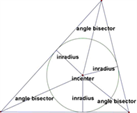#### 5) Euler Line of a triangle:

It is the line which passes through the centroid, circumcenter, and orthocenter.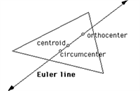### Types of Triangles:

1) Based on type of angle

2) Based on the length of side

### A right triangle is a triangle with a right angle (i.e. 90°).

The side opposite the right angle is always the triangle’s longest side. It is called the hypotenuse of the triangle. The other two sides are called the legs.

The lengths of the sides of a right triangle are related by Pythagorean Theorem.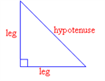Example 1:

A right triangle has one other angle that is 35º. What is the size of the third angle?

Solution:

Step 1: A right triangle has one angle = 90°. Sum of known angles is 90° + 35º = 125°.

Step 2: The sum of all the angles in any triangle is 180º.

Subtract the sum of known angles from 180°.

180° – 125° = 55°

Answer: The size of the third angle is 55°

#### Note:- Median to the hypotenuse= ½ * hypotenuse = Circumradius of the triangle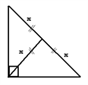#### b) Acute Triangles

An acute triangle is a triangle whose angles are all acute (i.e. less than 90°). In the acute triangle shown below, a, b and c are all acute angles.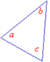Example Question:

The lengths of the sides of a triangle are 10,24 and x, where x is a positive integer. Find the values of “x” for which this triangle has 3 acute angles.

Solution:

In an acute angled triangle; the sum of the squares of any two sides of a triangle have to be greater than the third side

i.e. 102+242>x2 ; x2+102242 ;and >x2+>242>102 computing the minimum and maximum “x”, we get the range as 26>x>v476. the values of “x” which satisfy the above conditions are 22,23,24 and 25.

#### c) Obtuse Triangles

An obtuse triangle has one obtuse angle (i.e. greater than 90º). The longest side is always opposite the obtuse angle. In the obtuse triangle shown below, a is the obtuse angle.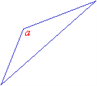#### Is it possible for a triangle to have more than one obtuse angle?

Step 1: Let the angles of the triangle be a, b and c. Let a be the obtuse angle.

Step 2: The sum of all the angles in any triangle is 180o. a + b + c = 180o.

If a> 90o then b + c must be less than 90o. Therefore, b and c must be acute angles. Thus, a triangle can only have one obtuse angle.

Note: In a right angled triangle with legs “a, “b” and “c”

a2+b2=c2

For an acute angled triangle,

=> a2+b2>c2

and for an obtuse-angled triangle,

=> a2+b2<c2

### 2) Different Triangles Based on length of side:

#### a) Equilateral Triangles

An equilateral triangle has all three sides equal in length. It’s three angles are also equal and they are each 60o.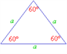Question:

An equilateral triangle has one side that measures 5 cm. What is the size of the angle opposite that side?

Solution:

Step 1: Since it is an equilateral triangle all its angles would be 60º. The size of the angle does not depend on the length of the side.

Answer: The size of the angle is 60º. Special Properties of Equilateral triangles

1) Height (h) = (v3)/2*side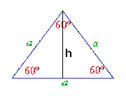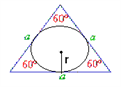3) Circumradius (R)= 2/3*height = side/(v3)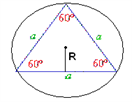Area= (v3)/4×(side)2

Perimeter (Sum of sides of the triangle)= 3×side

An equilateral triangle has maximum area. In an equilateral triangle, the median, angle bisector, and altitude are equal. Also, they intersect at point G, the center of the inscribed and circumscribed circle as shown below.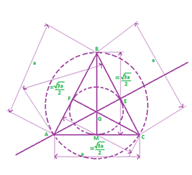Question:

P is the point of intersection of the three angle bisectors of equilateral triangle ABC with side “a”. What is the length of AP?

Solution:

P is nothing but the incentre of this triangle. AP= 1/3 ×v3/2 a 2/3*(v3)/2a=a/(v3)

b) Isosceles Triangles An isosceles triangle has two sides of equal length. The angles opposite the equal sides are also equal.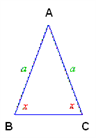Question:

An isosceles triangle has one angle of 96 o. What are the sizes of the other two angles?

Solution:

Step 1: Since it is an isosceles triangle it will have two equal angles. The given 96º angle cannot be one of the equal pair because a triangle cannot have two obtuse angles

Step 2: Let x be one of the two equal angles. The sum of all the angles in any triangle is 180°. x + x + 96° = 180° 2x = 84° =>x = 42° Answer: The sizes of the other two angles are 42o each.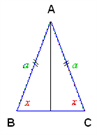#### b) Scalene Triangles:

A scalene triangle has no sides of equal length. Its angles are also all different in size.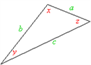i.e. here a?b?c and x?y?z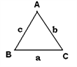Note:-* In a triangle ABC; Semi perimeter (s)= (a+b+c)/2

### Formulae to Find the Area of a Triangle

1) Area of a triangle = v(s(s-a)(s-b)(s-c) )

(“s” is semiperimeter and a,b,c are the sides of the triangle)

2) Area of a triangle= 1/2bc*sinA= 1/2ab*sinC= 1/2ac*sinB

3) Area of a triangle= abc/4R

4) Area of a triangle= r*s

(“r” is the inradius and “s” is the semiperimeter)

Areas of two triangles having the same base and lying between the same parallel lines will be equal.

In this figure, Area of ABC= Area of PBC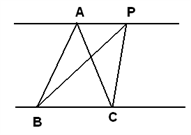ILLUSTRATION

7) In ?PQR, PQ=5cm, QR= 6 sm, and PR=7 cm. A point X, inside the triangle is at a distance of 2 cm from PQ and 3 cm from QR. Find the distance between X and PR Solution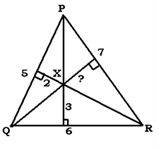Area of a triangle =v(s(s-a)(s-b)(s-c)) (s=[5+6+7]/2=9)

Area= v((9x4x3x2))= 6v6 cm

Area of PQR can also be written as (Area of ? PXQ + ?PXR +?QXR)

6v6 = (½ x2x5) + (1/2x3x6)+(1/2x7xp)

Solving, we get p=(12v6- 28)/7

TESTS OF SIMILARITY

Similar triangles have the following properties:

• They have the same shape but not the same size.

• Each corresponding pair of angles is equal.

• The ratio of any pair of corresponding sides is the same.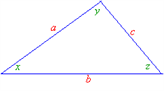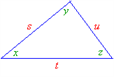If the above two triangles are similar then

a/s=b/t=c/u

When the ratio is 1 then the similar triangles become congruent triangles (same shape and size).

We can tell whether two triangles are similar without testing all the sides and all the angles of the two triangles. There are two rules to check for similar triangles.

1) AA rule

2) SAS rule

As long as one of the rules is true, it is sufficient to prove that the two triangles are similar.

1) AA Rule

If two angles of one triangle are equal to two angles of another triangle, then the triangles are similar.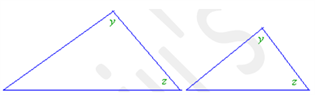Example 1: Given the following triangles, find the length of s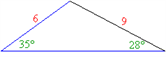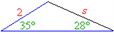Solution:

The ratios of the lengths are equal.

6/2=9/S

Cross multiplying: 6s = 18 =>s = 3

The length of s is 3

2) SAS Rule

If two sides of two triangles are in the same ratio and their included angle is equal, then the triangles are similar.

An included angle is the angle formed by the two given sides.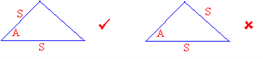Included Angle Non-included angle

For example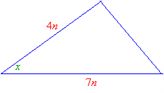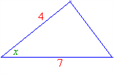Example 2: Given the following triangles, find the length of s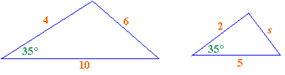Solution:

The ratios of the lengths are equal.

4/2=6/S

4S=12

S=3

Answer: The length of s is 3

Tests of Congruency

Congruent triangles are triangles that have the same size and shape. This means that the corresponding sides are equal and the corresponding angles are equal.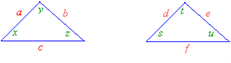In the above diagrams, the corresponding sides are a and d; b and e ;c and f.

The corresponding angles are x and s; y and t; z and u.

There are four rules to check for congruent triangles.

1) SSS rule

2) SAS rule

3) ASA rule and

4) AAS rule.

1) SSS Rule

If three sides of one triangle are equal to three sides of another triangle, then the triangles are congruent. (Hence the name of this rule: Side-Side-Side, SSS)

2) SAS Rule

If two sides and the included angle (Side-Angle-Side, SAS) of one triangle are equal to two sides and included angle of another triangle, then the triangles are congruent.

3) ASA Rule

If two angles and the included side of one triangle (Angle-Side-Angle, ASA) are equal to two angles and included side of another triangle, then the triangles are congruent. An included side is the side between the two given angles.

4) AAS Rule

If two angles and a non-included side of one triangle (Angle-Angle-Side, AAS) are equal to two angles and a non-included side of another triangle, then the triangles are congruent. (This rule may sometimes be refered to as SAA). For the ASA rule the given side must be included and for AAS rule the side given must not be included. The trick is we must use the same rule for both the triangles that we are comparing.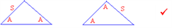Comparing AAS with AAS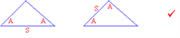Comparing ASA with ASA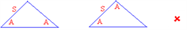Comparing AAS with ASA

Important Theorems

1) Theorem 1-The Pythagorean Theorem

It is a formula relating the lengths of the three sides of a right triangle. It is used when we know the length of two sides of a right triangle and we need to get the length of the third side.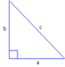If we take the length of the hypotenuse to be c and the length of the legs to be a and b then this theorem tells us that:

c2 =a2 + b2

SPECIAL RIGHT ANGLED TRIANGLES

A) 3–4–5 Triangles

A 3-4-5 triangle is right triangle whose lengths are in the ratio of 3:4:5.This is nothing but an application of Pythagoras theorem

Side1 : Side2 : Hypotenuse = 3n : 4n : 5n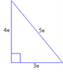8) Find the length of the hypotenuse of a right triangle if the lengths of the other two sides are 6 inches and 8 inches.

Solution:

1. Test the ratio of the lengths to see if it fits the 3n : 4n : 5n ratio. 6 : 8 : x = 3(2) : 4(2) : x

2. It is a 3-4-5 triangle for n = 2.

3.Calculate the third side 5n = 5×2 = 10

The length of the hypotenuse is 10 inches.

B) 45º-45º-90º Triangles

A 45o– 45o– 90o triangle is a triangle whose angles are 45o, 45oand 90o. The lengths of the sides of a 45o – 45o – 90o triangle are in the ratio of 1:1:2 .

A right triangle with two sides of equal lengths is a 45o– 45o– 90o triangle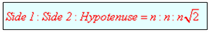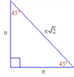9) Find the length of the hypotenuse of a right triangle if the lengths of the other two sides are both 3 inches.

Solution:

1: This is a right triangle with two equal sides so it must be a 45°- 45°- 90° triangle.

2: You are given that the both the sides are

3. If the first and second value of the ratio n:n:n2 is 3 then the length of the third side is 32

Answer: The length of the hypotenuse is inches.

A right triangle with a 45° angle must be a 45°- 45°- 90° right triangle.

3) 30o-60o-90o Triangles

This is right triangle whose angles are 30o, 60o and 90o. The lengths of the sides of a 30o – 60o – 90o triangle are in the ratio of 1:3:2

Side 1: Side 2:Hypotenuse=n:n3:2n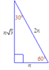A right triangle with a 30° angle or 60° angle must be a 30°- 60°- 90° triangle.

10) Find the lengths of the other two sides of a right triangle if the length of the hypotenuse is 8 inches and one of the angles is 30°.

This is a right triangle with a 30° angle so it must be a 30°- 60°- 90° triangle. You are given that the hypotenuse is 8.

Substituting 8 into the third value of the ratio n:n3:2n , we get that 2n = 8 i.e.n = 4. Substituting n = 4 into the first and second value of the ratio we get that the other two sides are 4 and 43. Answer:

The lengths of the two sides are 4 inches and 43 inches.

Theorem 2- Areas and Similarity

Ratio of Areas of 2 similar triangles = Ratio of squares of the corresponding sidesIf ?ABC ˜ ?PQR

Then Area(?ABC)/Area(?PQR) =(AB2)/(PQ2 )=(BC2)/(QR2 )=(AC2)/(PR2 )

11) In triangle ABC, the line segment XY intersects AB In X and AC in Y, such that XY is parallel to BC. Find the ratio of XB:XA if it is known that the area of triangle AXY is half that of ABC

a) v2/(v2-1) b) (v2-1) c) ((v2+1))/v2 d) ((v2+2))/v2

Solution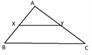Given that A(AXY)/A(ABC) =1/2

Thus ratio of the sides = 1/v2

Thus, XY/BC=AX/AB=AY/AC=1/v2

Thus, required ratio of XB: AX= ((v2-1))/1

Theorem 3-Mid Point Theorem

The segment joining the midpoints of any two sides of a triangle is parallel to the third side and is half the third side.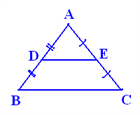Here if AD=DB and AE=EC, then DE is parallel to BC and DE=1/2 BC

Theorem 4- Proportionality Theorem

If a line is drawn parallel to one side of a triangle and intersects the other two sides in two distinct points, then the other sides are divided in the same ratio by it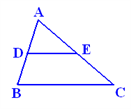Here AD/DB=AE/EC where DE is parallel to BC

Theorem 5- Interior Angle Bisector Theorem

The angle bisector of any angle of a triangle divides the side opposite to the angle in the ratio of the remaining two sides.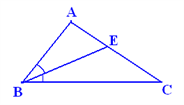In ?ABC, if BE is the angle bisector of ABC, then BA/BC=AE/CE

12) AM is the median of ?ABC. The bisectors of Let YC= p From the interior angle bisector theorem AM/MB=AX/XB=> 1:4 Since BM=MC (AM is the median) AM:MC= 1:4 = 2:p =>p= 8 Thus AC =10 cm

#### Theorem 6- Exterior Angle Bisector Theorem

The angle bisector of any angle of a triangle divides the side opposite to the angle in the ratio of the remaining two sides.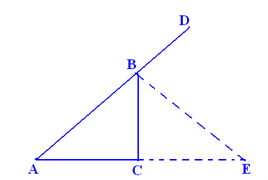In ?ABC, If BE is the angle bisector of ABC, then ,BA/BC=AE/CE

Theorem 7- Appollonius theorem

The sum of the squares of any two sides of a triangle is equal to the twice of the sum of the square of the median to the third side and the square of half the third side